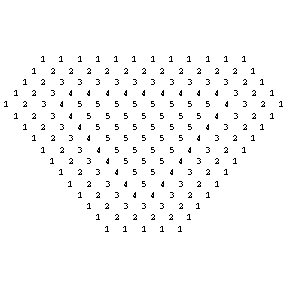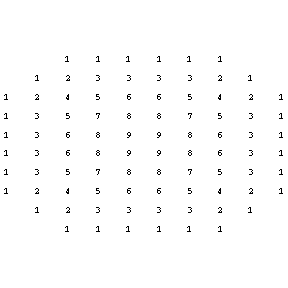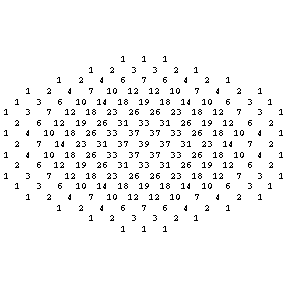## Mathematica Code to compute Weight diagrams## Mathematica

Availability Mathematica is installed on some computers in the basement of the Science center. It might also be installed on some computers in the houses. If you want to try an installation of mathematica on your own computer, get it here. Note that you have to be on a Harvard network and have your PIN ready to download the software and that requesting the Mathematica Password requires you to send the request from a Harvard computer.
Installation After you downloaded the program to your computer, start the program and follow the instructions. During the installation progress, you have to enter the Harvard Licence number L2482-2405. The number which you will get in return has to be entered in the Mathematica Registration page. You will then be sent a password by email. This is what you see during installation in
Running mathematica Mathematica is started like any other application on Macintoshs or PC's. On Linux, just type "mathematica" to start the notebook version, or "math" to start the terminal version.
Some frequently used commands: ( See more what you can do with Mathematica)
 N[Pi,1000] Get Pi to 1000 digits Eigenvalues[{{1,2,3},{3,2,1},{1,2,3}}] Compute the eigenvalues of a matrix Eigensystem[{{1,2,3},{3,2,1},{1,2,3}}] Find the eigensystem of a matrix Solve[x^2+3x+4==0,x] Solve an equation NSolve[x^2+3x+4==0,x] Solve an equation numerically Det[{{1,2,3},{3,2,1},{1,2,6}}] Find the determinant of a matrix Plot[ x Sin[x],{x,-10,10}] Graph function of one variable Plot3D[ Sin[x y],{x,-2,2},{y,-2,2}] Graph function of two variables ParametricPlot[ {Cos[3 t],Sin[5 t]} ,{t,0,2Pi}] Plot planar curve ParametricPlot3D[ {Cos[t],Sin[t],t} ,{t,0,4Pi},AspectRatio->1] Plot space curve ParametricPlot3D[ {Cos[t] Sin[s],Sin[t] Sin[s],Cos[s]},{t,0,2Pi},{s,0,Pi}] Parametric Surface ContourPlot[ Sin[x y],{x,-2,2},{y,-2,2} ] Contour lines (traces) Integrate[ x Sin[x], x] Integrate symbolically NIntegrate[ Exp[-x^2],{x,0,10}] Integrate numerically D[ Cos^5[x],x ] Differentiate symbolically Series[Exp[x],{x,0,3} ] Taylor series DSolve[ x''[t]==-x[t],x,t ] Solution to ODE Get["Graphics`ContourPlot3D`"]; ContourPlot3D[x^2+2y^2-z^2-1,{x,-2,2},{y,-2,2},{z,-2,2}] Implicit surface